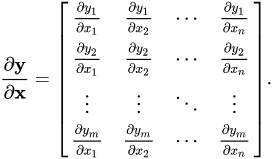# Matrices in Calculus

Examples are a very important tool in understanding mathematics, including linear maps and matrices. Common examples of linear maps include differentiation and integration where c = 0 as maps between vector spaces of polynomials. Through experience with these operations, it is clear to see that each follows the properties of additivity and homogeneity. Let D represent differentiation and I represent integration, the properties mean that D(a+b) = D(a) + D(b) and cD(a) = D(ca) for a constant c.

For some degree polynomial, n, the derivative maps $$x^n$$ to $$n*x^{n-1}$$ and the integral maps $$x^n$$ to $$\frac{x^{n+1}}{n+1}$$. This way of expressing the operations draws the connection to the basis elements of the space of polynomials. Since we have already defined the maps on the basis elements, a matrix can be constructed for both operations. The general construction of these matrices will be the coefficients (1, 2, 3 … and 1, ½, ⅓…) along the diagonal to represent multiplying or dividing by the power shifted to represent the change in power.These matrices, or at least the infinitely large varieties, can be applied to any polynomial to yield the derivative or integral. The way this would be done with matrix operations is by multiplying the matrix for the operation by the n-by-1 matrix representing the input function.

Since the integral and derivative are inverse functions of one another, the composition of the functions yields the original function, meaning that the product of the matrices representing integration and differentiation will be the identity matrix, as seen below.

This example is a good way to understand the notion of how linear maps are represented as matrices because of experience with derivatives and integrals already relying on the necessary properties of linear maps.

Another matrix in calculus is the total derivative of a function, which is a linear map from $$R^n$$ to $$R^m$$ based on a function between the two sets. The total derivative of a function is a set of linear maps between the basis elements of the domain and the rate of change of a particular element of the basis of the codomain in that direction.Under the condition that all the partial derivatives exist, the total derivative corresponds to the Jacobian matrix, which is used to transform spaces in vector calculus.

The exact transformation created by the Jacobian matrix is left as an important exercise to those studying vector calculus.

Contributor: Joseph Brewster